# How to implement Dictionary with Python3?

• Difficulty Level : Expert
• Last Updated : 04 Dec, 2019

This program uses python’s container called dictionary (in dictionary a key is associated with some information). This program will take a word as input and returns the meaning of that word.
Python3 should be installed in your system. If it not installed, install it from this link. Always try to install the latest version.

I made a text file in which word and its meaning is stored in python’s dictionary format
Example :

```data = {"geek" : "engage in or discuss computer-related tasks
obsessively or with great attention to technical detail."}
```

Here if we call “geek” from data then this will return its meaning “engage in or discuss computer-related tasks obsessively or with great attention to technical detail.”.

This python program allow you to fetch the data of this text file and give the meaning.

 `# Python3 Code for implementing``# dictionary`` ` `# importing json library``import` `json`` ` `# importing get_close_matches function from difflib library``from` `difflib ``import` `get_close_matches`` ` `# loading data``data ``=` `json.load(``open``(``"data.txt"``))`` ` `# defining function meaning``def` `meaning(w):`` ` `    ``# converting all the letters of "w" to lower case``    ``w ``=` `w.lower()`` ` `    ``# checking if "w" is in data``    ``if` `w ``in` `data:``        ``return` `data[w]`` ` `    ``# if word is not in data then get close match of the word``    ``elif` `len``(get_close_matches(w, data.keys())) > ``0``:`` ` `        ``# asking user for his feedback``        ``# get_close_matches returns a list of the best ``        ``# “good enough” matches choosing first close ``        ``# match "get_close_matches(w, data.keys())"``        ``yn ``=` `input``("Did you mean ``%` `s instead? Enter Y ``if` `yes, ``or` `N ``if` `no:``        ``" ``%` `get_close_matches(w, data.keys())[``0``])``         ` `        ``if` `yn ``=``=` `"Y"``:``            ``return` `data[get_close_matches(w, data.keys())[``0``]]``        ``elif` `yn ``=``=` `"N"``:``            ``return` `"The word doesn't exist in our data."``        ``else``:``            ``return` `"We didn't understand your entry."``    ``else``:``        ``return` `"The word doesn't exist in our data."`` ` `# asking word from user to get the meaning``word ``=` `input``(``"Enter word: "``)`` ` `# storing return value in "output"``output ``=` `meaning(word)`` ` `# if output type is list then print all element of the list``if` `type``(output) ``=``=` `list``:``    ``for` `item ``in` `output:``        ``print``(item)`` ` `# if output type is not "list" then print output only``else``:``    ``print``(output)`

How to run?

1. Download this data file and save it in the same folder where your python code file is saved.
2. Make sure that both the file(data file and the code file) are in same folder.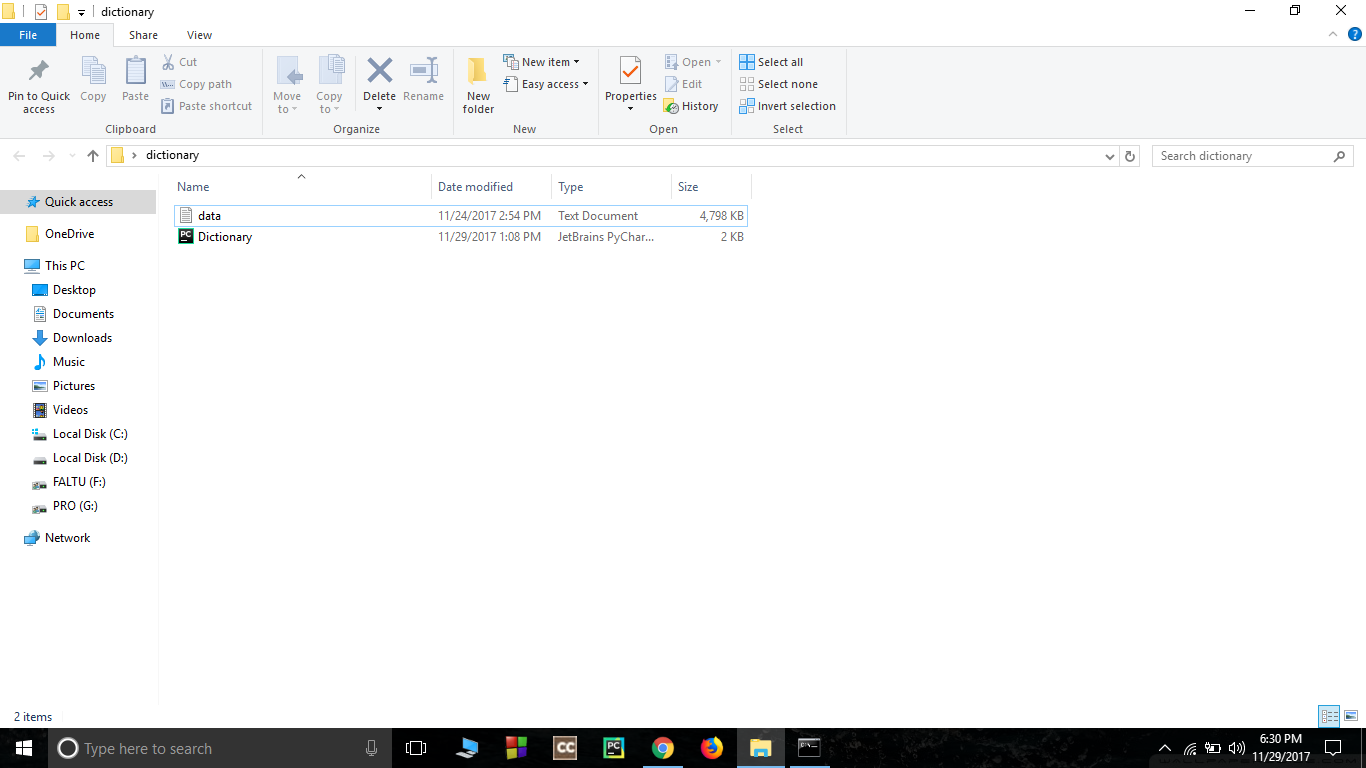3. Open command prompt in that folder to do so press shift then right click in mouse.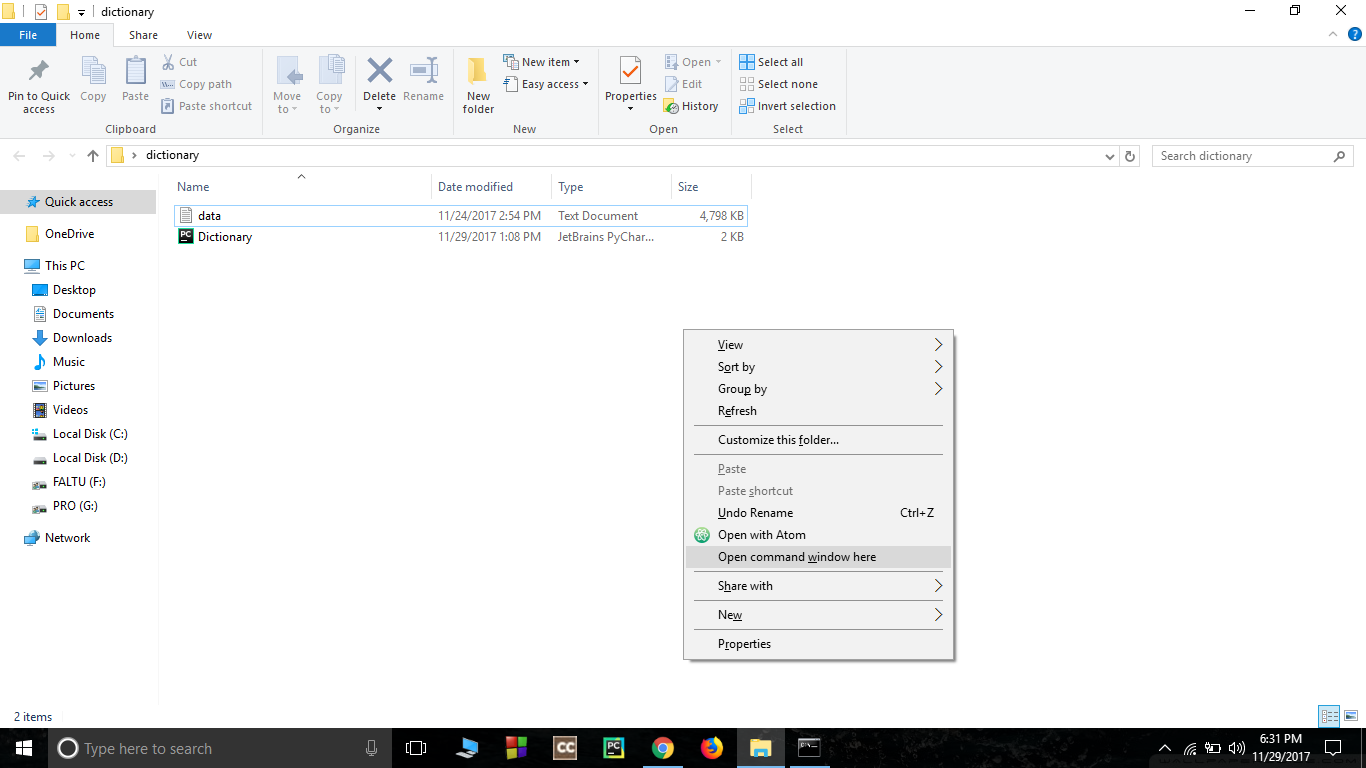4. Run the python code using cmd(command prompt).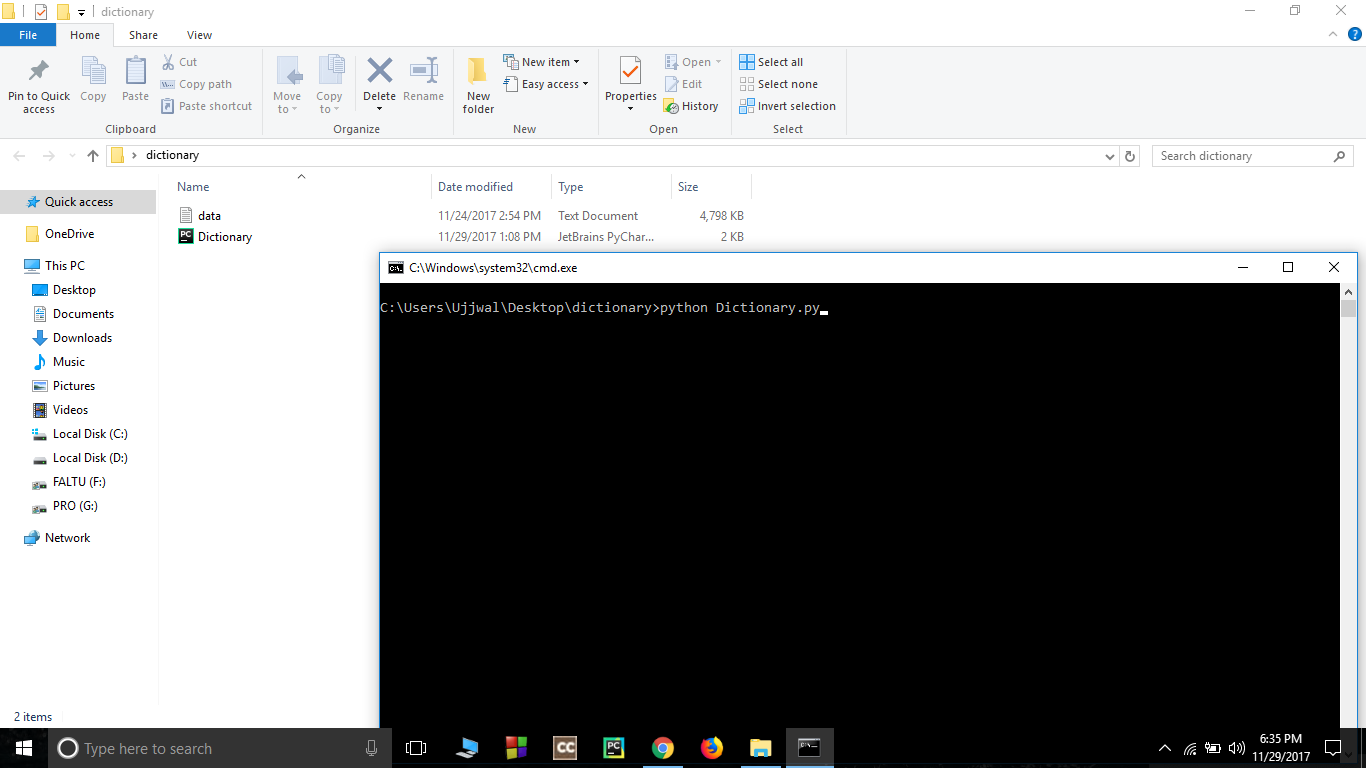5. Input the word whose meaning is to be searched.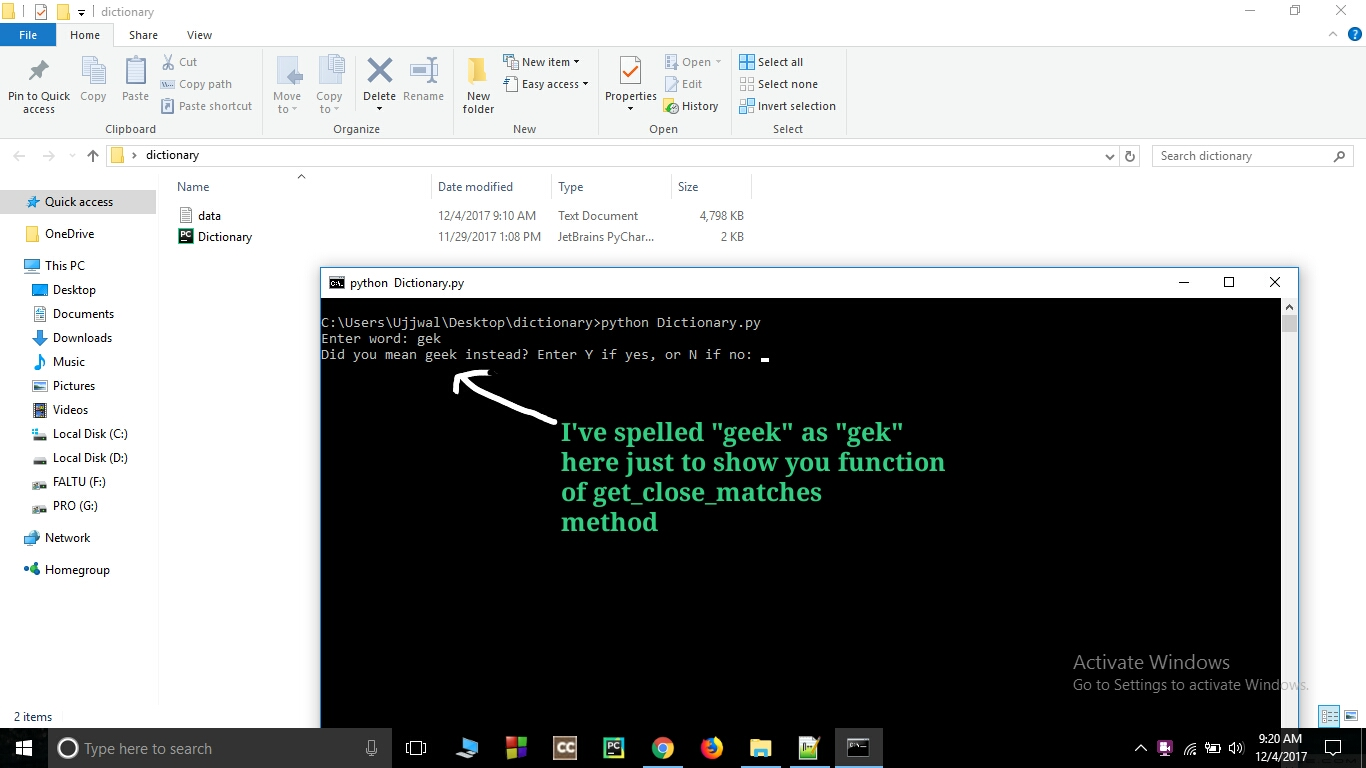6. Output will be your result.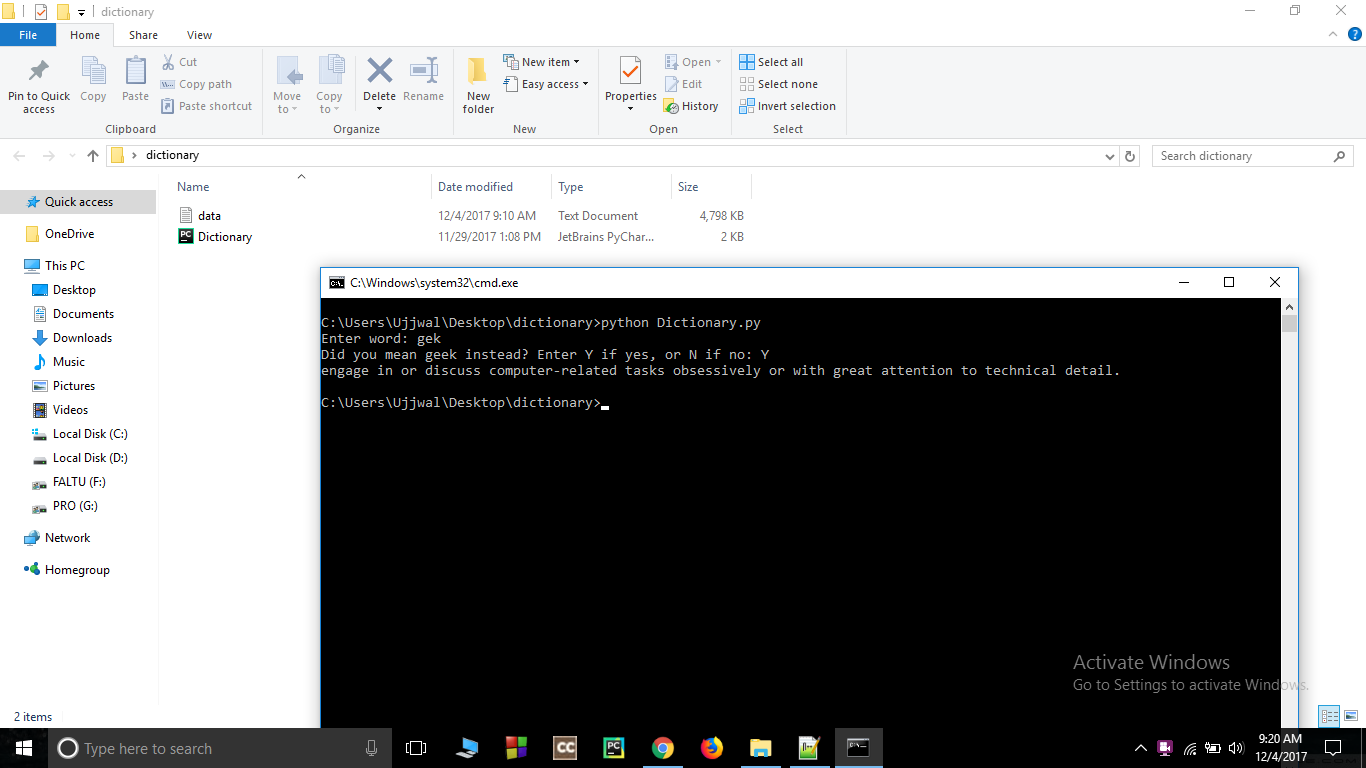Video Demonstration

My Personal Notes arrow_drop_up